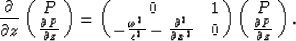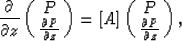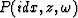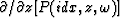Next: Horizontally Uniform Structures Up: Ji: generalized phase-shift method Previous: Introduction

# THE GENERALIZED PHASE-SHIFT METHOD

The generalized phase-shift method is based on the solution of the temporally transformed acoustic wave equation(1)
wheredenotes the temporal transform of the pressure field and c(x,z) is velocity field. As suggested by Kosloff and Baysal(1983), it is convenient to recast equation (1) as a set of two first-order coupled equations given by(2)
The downward continuation in the migration consists of the solution of equation (2) for each frequency at all depths under the initial conditions of the values of P andat the surface z=0 (Kosloff and Baysal, 1983). Equation (2) can be expressed in a more compact form(3)
wheredenotes a column vector of length 2Nx containing first the Nx pressuresand then the Nx pressure derivatives, for i=0,...,Nx-1. As with the ordinary phase-shift method, the solution here is propagated in depth increments. Within each increment z to z+dz, the velocity is assumed to be invariant in the vertical direction although it may vary horizontally. The solution of equation (3) can then be written as(4)
The solution (4) embodies a phase-shift of the eigenvector coefficients of A. It would therefore seem that a matrix diagonalization would have to be performed before each propagation.Next: Horizontally Uniform Structures Up: Ji: generalized phase-shift method Previous: Introduction
Stanford Exploration Project
1/13/1998